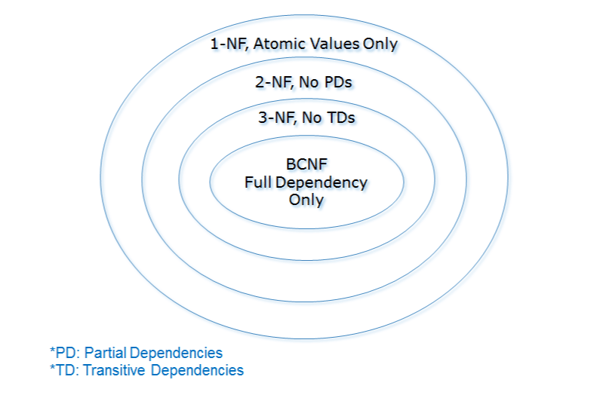Related Tags

bcnf
normalization
normalform
communitycreator

# What is Boyce-Codd normal form (BCNF)?

Fatima Hasan

## Normal forms

The process of normalization refers to the reduction of redundancy from a set of relations. In database tables, we can use normal forms to carry out this process. The four main normal forms are:

As the figure below shows, these normal forms build upon one another progressively. In this shot, we will focus on the Boyce-Codd normal form.## BCNF

For a relation to be in BCNF, it needs to satisfy the following conditions:

• It needs to be in third normal form.
• For every functional dependency X -> A, X should be a superkey.a single column or set of columns which uniquely identify a row

## Example

A B C
A1 B1 C1
A1 B2 C2
A2 B1 C1
A3 B3 C3

In the above relation, AB is the key. However, there is a functional dependency, as follows:

### C -> B

The relation is in the first three normal forms because:

• All column values are atomic and have the same data type.
• There is no partial dependency.
• There is no transitive dependency.

However, the table is not in BCNF because in the dependency given above, C is not a superkey.

To achieve BCNF, we will have to remove the given dependency by splitting the table into two, as follows:

A B
A1 B1
A1 B2
A2 B1
A3 B3
C B
C1 B1
C2 B2
C3 B3

The first table has AB as the key. The second table has C as the key. There are no dependencies X->A where X is not a superkey. Hence, the relation is now in BCNF.

An algorithm to convert any relation to BCNF is given below.

1. R = { R0 }
2. While there is a relation in R which is not in BCNF:
2.1. Choose a relation in R which is not in BCNF
2.2. Find a dependency X->Y which is not in BCNF
2.3. Decompose the relation into two relations as R1 = X+ and R2 = [ X U A ], where A is the attributes not in X+

RELATED TAGS

bcnf
normalization
normalform
communitycreator

CONTRIBUTOR

Fatima Hasan
RELATED COURSES

View all Courses

Keep Exploring

Learn in-demand tech skills in half the time